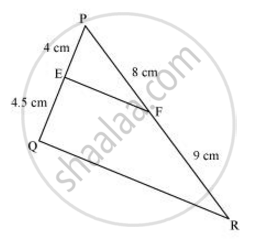# E and F are points on the sides PQ and PR respectively of a ΔPQR. For the following case, state whether EF || QR - Mathematics

E and F are points on the sides PQ and PR respectively of a ΔPQR. For the following case, state whether EF || QR

PE = 4 cm, QE = 4.5 cm, PF = 8 cm and RF = 9 cm

#### SolutionPE = 4 cm, QE = 4.5 cm, PF = 8 cm, RF = 9 cm

(PE)/(EQ) = 4/4.5=8/9

(PF)/(FR) = 8/9

(PF)/(FR)=(PE)/(EQ)

Therefore, EF is parallel to QR

Concept: Similarity of Triangles
Is there an error in this question or solution?

#### APPEARS IN

NCERT Class 10 Maths
Chapter 6 Triangles
Exercise 6.2 | Q 2.2 | Page 128